Author: Oscar Cronquist Article last updated on February 27, 2019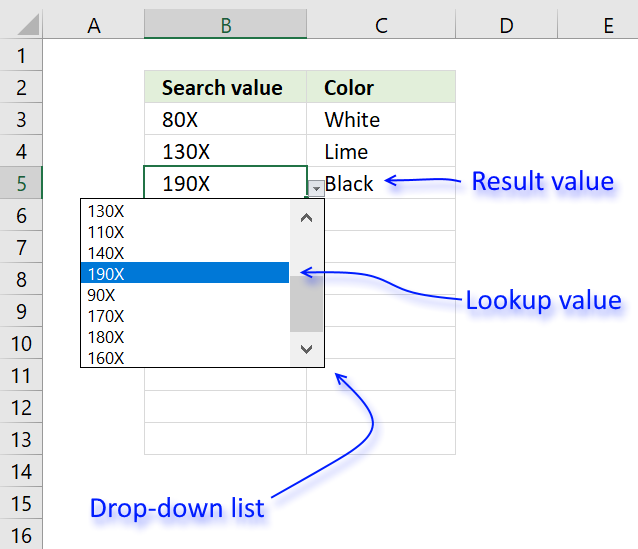I will in this article demonstrate how to use a value from a drop-down list and use it to do a lookup in a dataset or preferably in an Excel defined Table. In other words, this will auto populate other cells when selecting values in a drop-down list.

I will also demonstrate how to create, edit and add values to a drop-down list. There are several kinds of drop-down lists in Excel. Data validation and Combo Boxes. They have their advantages and disadvantages and I will discuss them in this article.

### Data validation drop-down list

The Data Validation drop-down list is easy to set up but it has its flaws, the purpose with data validation is to force the user to select one out of several predetermined values.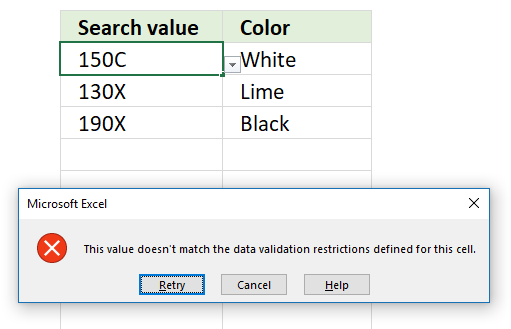This can, however, be easily ignored by the user simply by pasting a value to the drop-down list.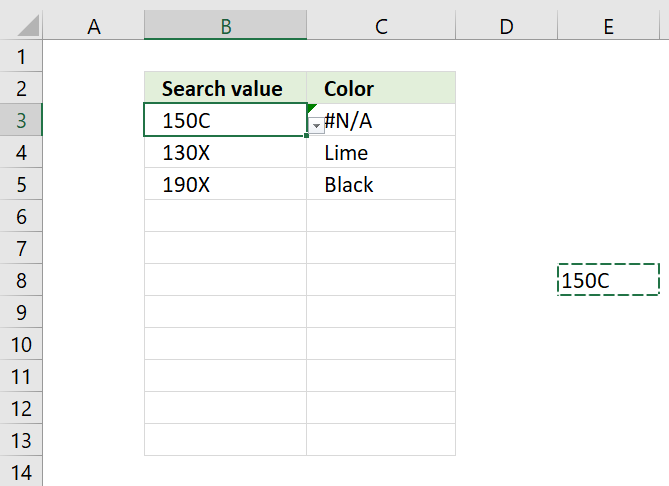Another disadvantage is that the data validation drop-down list won't allow you to search for a value in the list, this can make it time-consuming and ineffective to use if it contains lots of values.

Tip! Make sure you have the values sorted from A to Z in a drop-down list to make life easier for the user.

I recommend using a combo box if you want to be able to search a drop-down list. A combo box is also a drop-down list that you can easily create and manipulate.

#### Create a drop-down list

The data source is in this case an Excel defined Table which has its advantages. To be able to use it in a drop-down list a workaround is needed, the INDIRECT function makes it possible to reference the Table as a source.

1. Select cell B3.
2. Go to "Data" tab on the ribbon.
3. Press with left mouse button on "Data Validation" button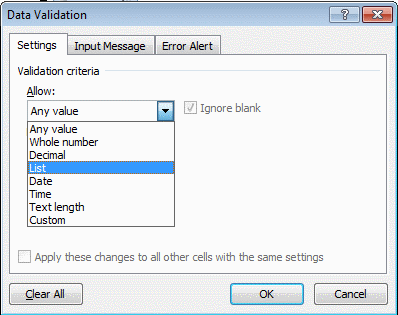4. Select List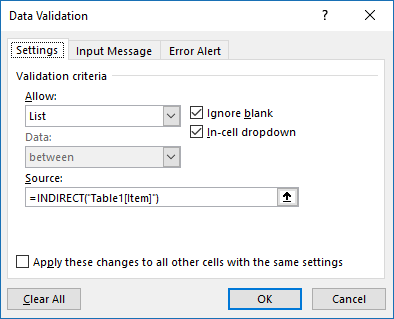5. Type =INDIRECT("Table1[Item]") in "Source:" window
6. Press with left mouse button on OK

### Formula that will auto populate a single cell

The first formula I will demonstrate is a simple INDEX - MATCH formula that will use the selected value in the drop-down list to search a dataset and return the adjacent value if a match is found.

Formula in cell C3:

=INDEX(Table1[Color], MATCH(Sheet1!\$B3, Table1[Item], 0))

The MATCH function returns the relative position of the drop-down list value in column Table1[Item] if found.

MATCH(Sheet1!\$B3, Table1[Item],0)

becomes

MATCH("80X", {"200X"; "120X"; "80X"; "70X"; "150X"; "100X"; "130X"; "110X"; "140X"; "190X"; "90X"; "170X"; "180X"; "160X"; "200X"; "120X"; "80X"; "150X"; "100X"; "130X"; "110X"; "90X"; "170X"; "180X"}, 0)

and returns 3. "80X" is found as the third value in the array.

Note that the MATCH function only returns the relative position of the first match.

The INDEX function returns the value in column Table1[Color] based on the relative position returned from the MATCH function.

INDEX(Table1[Color], MATCH(Sheet1!\$B3, Table1[Item], 0))

becomes

INDEX(Table1[Color], 3)

becomes

INDEX({"Navy"; "Lime"; "White"; "Yellow"; "Magenta / Fuchsia"; "Gray"; "Lime"; "Cyan / Aqua"; "Purple"; "Black"; "Teal"; "Magenta / Fuchsia"; "White"; "Teal"; "Teal"; "White"; "Magenta / Fuchsia"; "Black"; "Teal"; "Gray"; "Yellow"; "Purple"; "Lime"; "Cyan / Aqua"}, 3)

and returns "White in cell C3.

### Formula that will auto populate several cells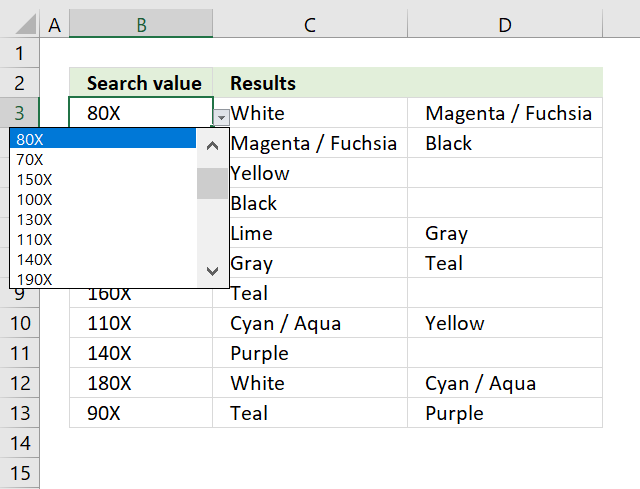The following formula is more complicated, it returns multiple values distributed horizontally starting from column C.

Array formula in cell C3:

=IFERROR(INDEX(Table1[Color], SMALL(IF(Sheet2!\$B3=Table1[Item], MATCH(ROW(Table1[Item]), ROW(Table1[Item])), ""), COLUMNS(\$A\$1:A1))), "")

To enter an array formula, type the formula in cell C3 then press and hold CTRL + SHIFT simultaneously, now press Enter once. Release all keys.

The formula bar now shows the formula with a beginning and ending curly bracket telling you that you entered the formula successfully. Don't enter the curly brackets yourself.

#### Explaining formula in cell C3

Step 1 - Find cells that equal condition

Sheet2!\$B3=Table1[Item] is a logical expression that returns boolean values, TRUE or FALSE.

Sheet2!\$B3=Table1[Item]

becomes

"80X"={"200X"; "120X"; "80X"; "70X"; "150X"; "100X"; "130X"; "110X"; "140X"; "190X"; "90X"; "170X"; "180X"; "160X"; "200X"; "120X"; "80X"; "150X"; "100X"; "130X"; "110X"; "90X"; "170X"; "180X"}

and returns this array:

{FALSE; FALSE; TRUE; FALSE; FALSE; FALSE; FALSE; FALSE; FALSE; FALSE; FALSE; FALSE; FALSE; FALSE; FALSE; FALSE; TRUE; FALSE; FALSE; FALSE; FALSE; FALSE; FALSE; FALSE}

Step 2 - Calculate row numbers of matching cells

The IF function has three arguments, the first one must be a logical expression. If the expression evaluates to TRUE then one thing happens (argument 2) and if FALSE another thing happens (argument 3).

The function replaces TRUE with the corresponding relative row number and FALSE with nothing "".

IF(Sheet2!\$B3=Table1[Item], MATCH(ROW(Table1[Item]), ROW(Table1[Item])), "")

becomes

IF({FALSE; FALSE; TRUE; FALSE; FALSE; FALSE; FALSE; FALSE; FALSE; FALSE; FALSE; FALSE; FALSE; FALSE; FALSE; FALSE; TRUE; FALSE; FALSE; FALSE; FALSE; FALSE; FALSE; FALSE}, MATCH(ROW(Table1[Item]), ROW(Table1[Item])), "")

becomes

IF({FALSE; FALSE; TRUE; FALSE; FALSE; FALSE; FALSE; FALSE; FALSE; FALSE; FALSE; FALSE; FALSE; FALSE; FALSE; FALSE; TRUE; FALSE; FALSE; FALSE; FALSE; FALSE; FALSE; FALSE}, {1; 2; 3; 4; 5; 6; 7; 8; 9; 10; 11; 12; 13; 14; 15; 16; 17; 18; 19; 20; 21; 22; 23; 24}, "")

and returns

{""; ""; 3; ""; ""; ""; ""; ""; ""; ""; ""; ""; ""; ""; ""; ""; 17; ""; ""; ""; ""; ""; ""; ""}.

Step 3 - Extract k-th smallest row number

To be able to return a new value in a cell each I use the SMALL function to filter column numbers from smallest to largest.

The COLUMNS function keeps track of the numbers based on an expanding cell reference. It will expand as the formula is copied to the cells below.

SMALL(IF(Sheet2!\$B3=Table1[Item], MATCH(ROW(Table1[Item]), ROW(Table1[Item])), ""), COLUMNS(\$A\$1:A1))

becomes

SMALL({""; ""; 3; ""; ""; ""; ""; ""; ""; ""; ""; ""; ""; ""; ""; ""; 17; ""; ""; ""; ""; ""; ""; ""}, COLUMNS(\$A\$1:A1))

becomes

SMALL({""; ""; 3; ""; ""; ""; ""; ""; ""; ""; ""; ""; ""; ""; ""; ""; 17; ""; ""; ""; ""; ""; ""; ""}, 1)

and returns 3.

Step 4 - Return value from Excel defined Table

The INDEX function returns a value based on a cell reference and column/row numbers.

INDEX(Table1[Color], SMALL(IF(Sheet2!\$B3=Table1[Item], MATCH(ROW(Table1[Item]), ROW(Table1[Item])), ""), COLUMNS(\$A\$1:A1)))

becomes

INDEX(Table1[Color], 3)

and returns "White" in cell C3.

Step 5 - Return blank if formula returns error

IFERROR(INDEX(Table1[Color], SMALL(IF(Sheet2!\$B3=Table1[Item], MATCH(ROW(Table1[Item]), ROW(Table1[Item])), ""), COLUMNS(\$A\$1:A1))), "")

The IFERROR handles all errors in a formula, it lets you specify a value to return if an error is found.

Note, the IFERROR function identiifes all errors, this may make it hard to spot errors. Use it with caution.

### Get the Excel fileUse-a-drop-down-list-to-search-and-return-multiple-values.xlsx